博客

2021-12-26 11:36:57 By F7487

前言

1. SATT ，即 Self-Adjusting Top Tree，是 2005 年 Tarjan 和 Werneck 在他们的论文《Self-Adjusting Top Trees》中提出的一种基于 Top Tree 理论的维护完全动态森林的数据结构。

2. 前置知识：Splay 。

3. 笔者第一次写博客，试图将 SATT 讲的通俗易懂，如果发现哪里讲错了也欢迎指出。

目录

$\texttt{1.问题引入 }$

$\texttt{2.树收缩}$

$\ \ \ \ \texttt{2.1 Compress \& Rake }$

$\ \ \ \ \texttt{2.2 簇}$

$\ \ \ \ \texttt{2.3 Top Tree }$

$\texttt{3. Self-Adjusting Top Tree }$

$\ \ \ \ \texttt{3.1 原理 }$

$\ \ \ \ \texttt{3.2 结构}$

$\texttt{4.代码实现}$

$\ \ \ \ \texttt{4.1 Push 类函数}$

$\ \ \ \ \texttt{4.2 Splay 类函数}$

$\ \ \ \ \texttt{4.3 Access 类函数}$

$\ \ \ \ \texttt{4.4 Link \& Cut}$

$\ \ \ \ \texttt{4.5 完整代码（Luogu\ P3690 【模板】动态树）}$

$\texttt{5.时间复杂度证明}$

$\ \ \ \ \texttt{5.1 势能分析}$

$\ \ \ \ \ \ \ \ \texttt{5.1-1 势能分析引入}$

$\ \ \ \ \ \ \ \ \texttt{5.1-2 Splay 复杂度证明}$

$\ \ \ \ \texttt{5.2 SATT 复杂度证明}$

$\texttt{6.例题}$

$\texttt{7.参考资料 }$

1.问题引入

• 删除，添加一条边，保证操作前后仍是一个森林。

• 整体修改某棵树上的某条简单路径（表现为将这条路径上的每个点都打上某个标记）。

• 整体修改以某个点为根的子树。

• 整体询问某棵树上的某条简单路径（如询问点的权值和）。

• 整体询问以某个点为根的子树。

2.树收缩

2.1 Compress & Rake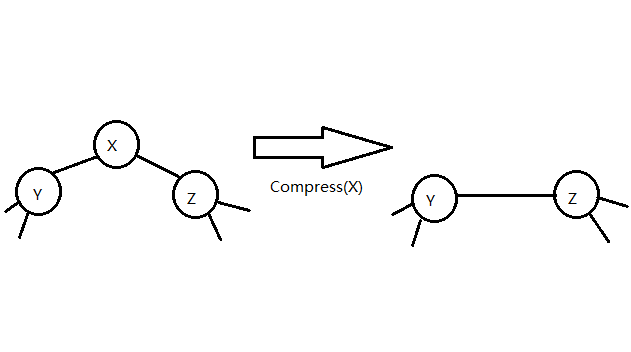Rake 操作指定一个度为一的点 $x$ ，这个点的邻点 $y$ 的度数需大于一，设点 $y$ 有一个邻点 $z (x \neq z)$，我们将点 $x$ ，边 $xy$ 的信息放入 边 $yz$ 中储存，并删去它们。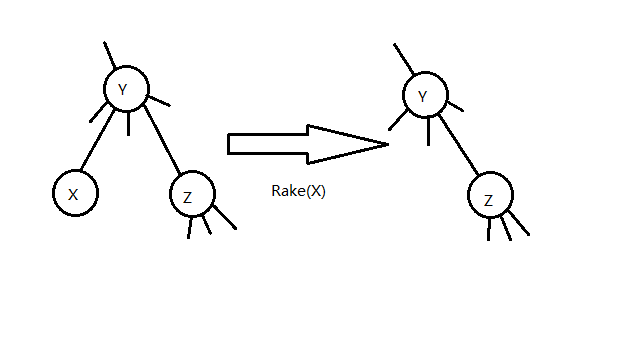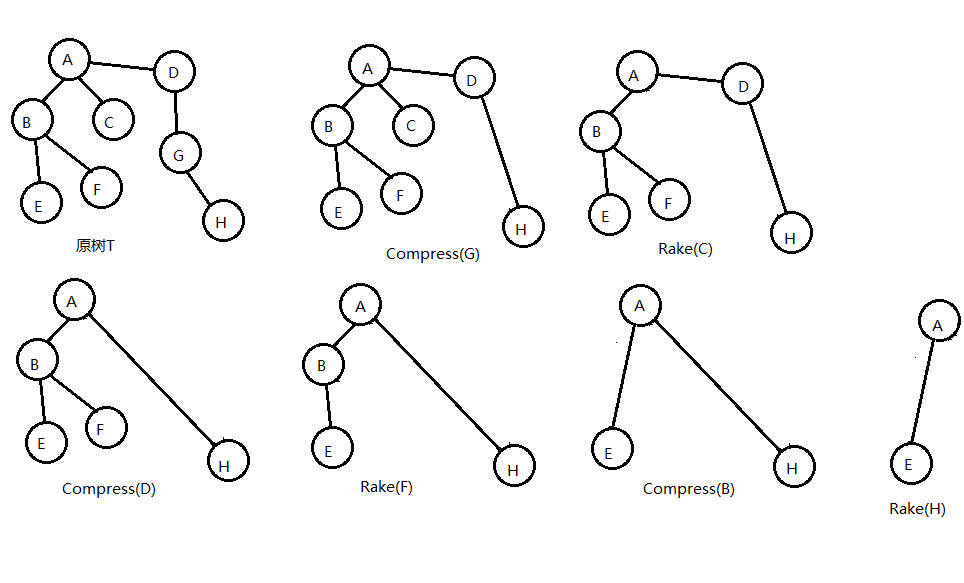2.2 簇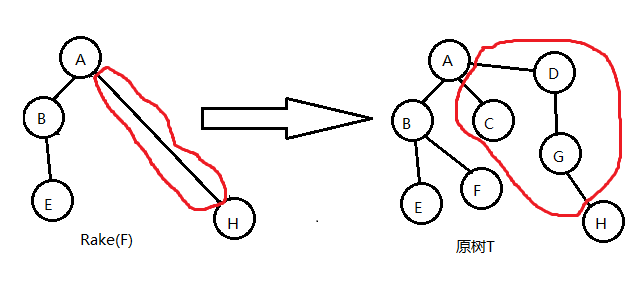1. 簇只维护内点和内边的信息。

2. 簇有两个端点。这两个端点即为 $T_x$ 中代表那个簇的边相连的那两个点。两个端点之间的路径我们称之为簇路径（cluster path） ；记两个端点分别为 $x$，$y$ ，我们用 $C(x,y)$ 来表示这个簇（在不同的 $T_x$ 中可能会有多个这样的簇）。

3. 内点仅与端点或内点相连，端点可能与非簇点相连。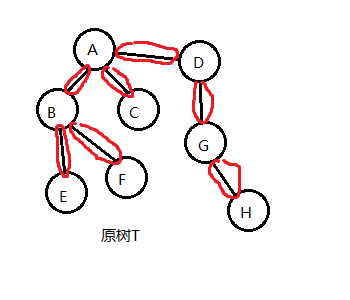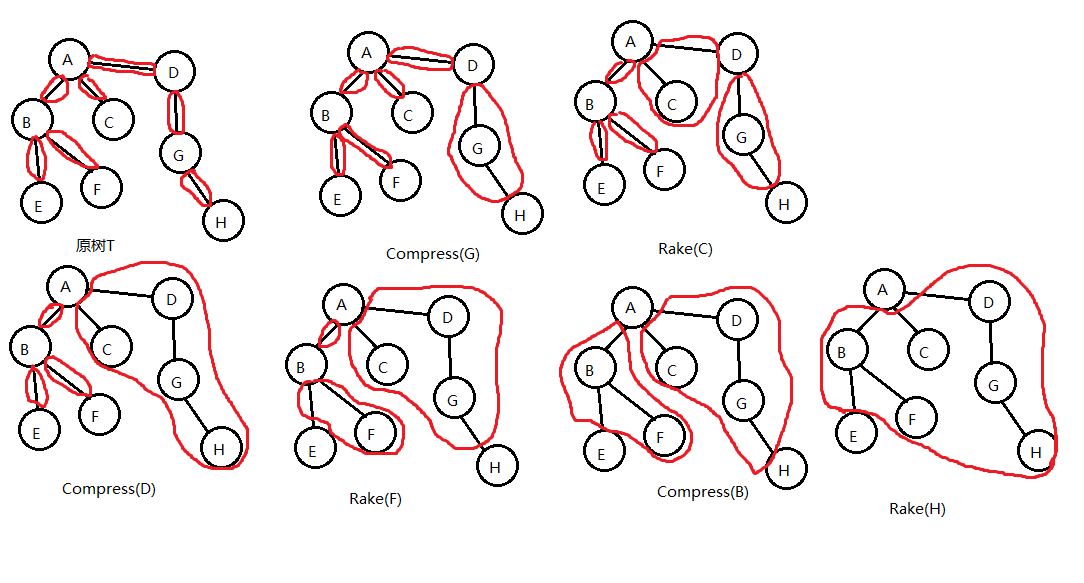2.3 Top TreeTop Tree 有以下性质;

1. 一棵 Top Tree 对应一棵原树和一种树收缩的方法，Top Tree 的每个节点都表示在某个 $T_x$ 中的某一条边，也就是树收缩过程中形成的某一个簇。图中的形如 $N_x$ 的点表示 $compress(x)$ （意义见 2-1）这一操作形成的簇。

2. Top Tree 中的一个节点有两个儿子（都分别代表一个簇），这个节点代表的簇是这两个簇通过 compress 或 rake 操作合并得到的新簇。

3. Top Tree 的叶子节点是基簇，其根节点是根簇。因此我们按一棵 Top Tree 的拓扑序分层，它的每一层就代表了一棵 $T_x$。

3.1 原理

Top Tree 对树收缩过程的极大简化 使我们看到通过维护树收缩过程来维护树上信息的可能性，SATT即是通过这一原理来维护树上信息的。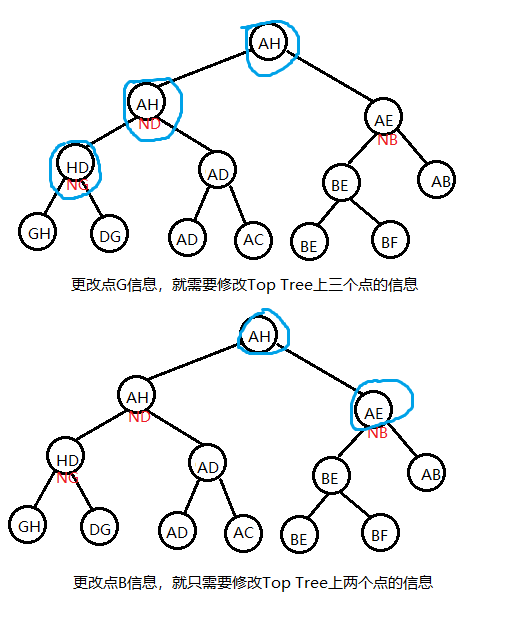SATT 就是通过修改 某个点/某条路径 在树收缩过程中信息被加入簇中的先后顺序来维护树上信息的。

3.2 实际结构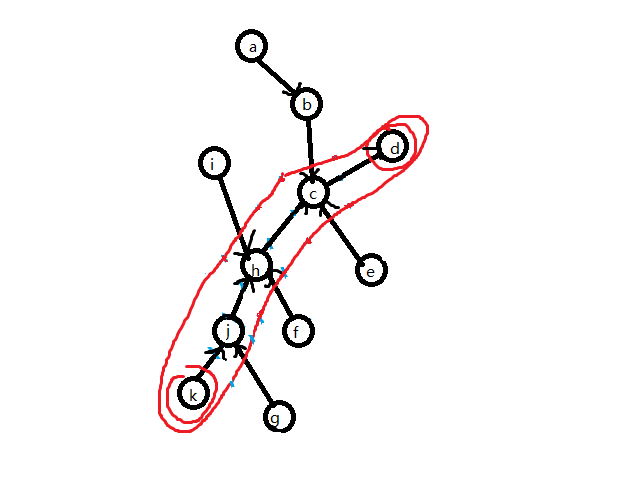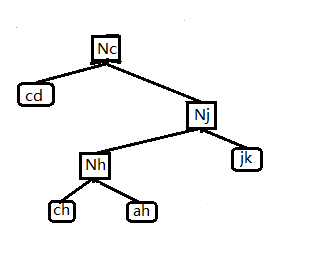compress tree 里的节点称为 compress node。只考虑当前这条簇路径，一个 非叶子的 compress node（必是 $compress(x)$ ） 就代表一次 compress 过程，表示将左儿子和右儿子信息合并起来，再将这个 $compress(x)$ 本身存储的点 $x$ 信息加入。这棵 compress tree 就维护了 $C(k,g)$ 簇路径的信息。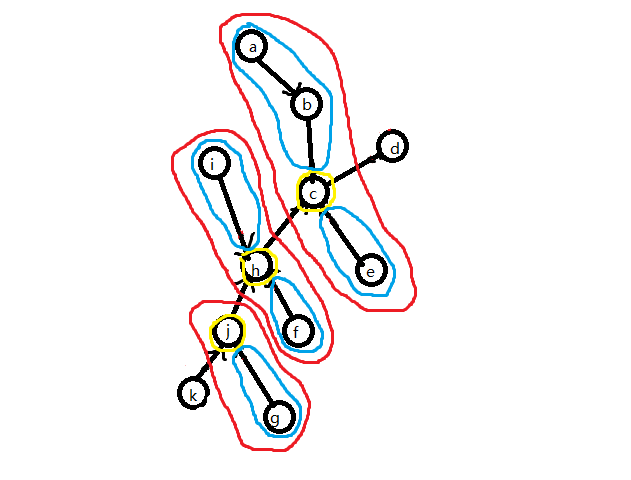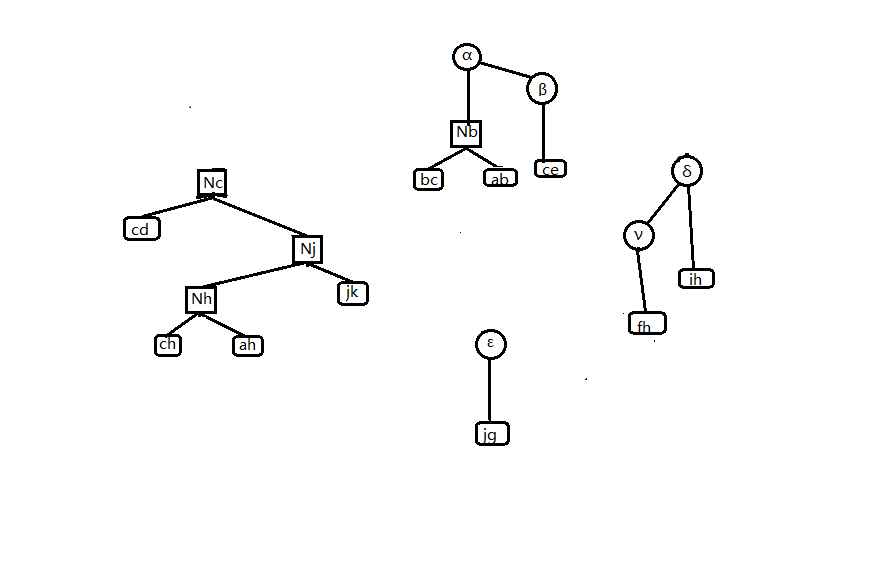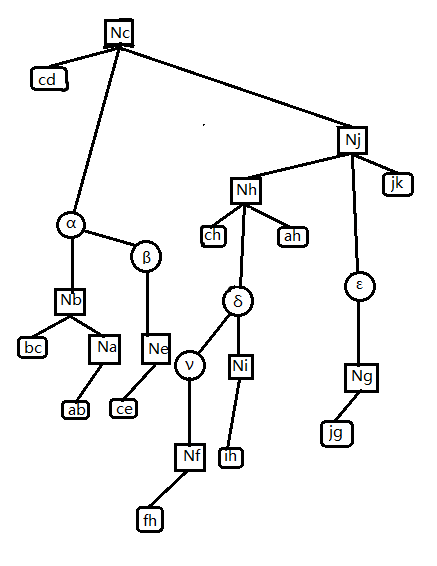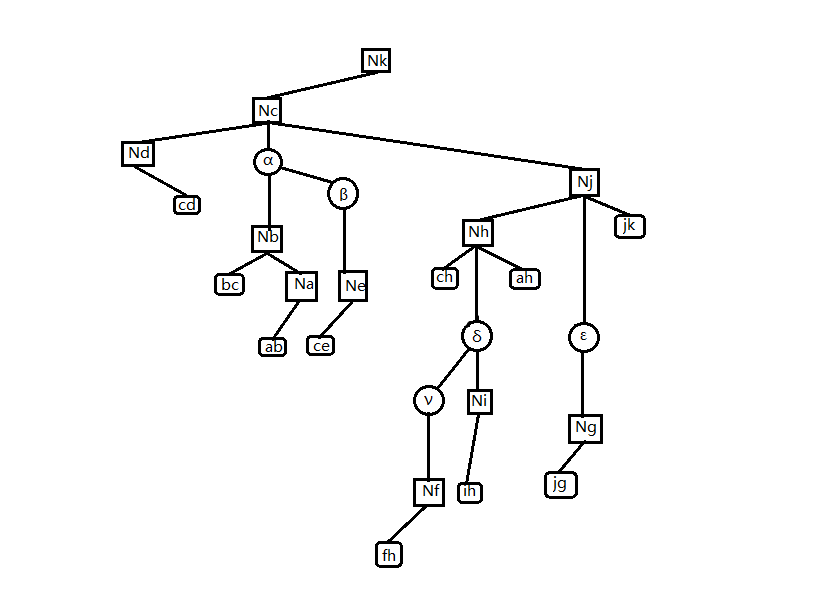1. SATT 由 compress tree 和 rake tree 组成，compress tree 是一棵特殊的 top tree；rake tree 是一个三叉树，它们都对应一棵树进行树收缩的过程。

2. compress node 里的点最多有三个儿子，非叶 compress node 可以任意旋转（保持 compress node 中儿子不变）。

3. rake tree 里的点一定有一个中儿子，rake node 可以任意旋转（保持 rake node 中儿子不变）。

4. SATT 的拓扑序反映了原树 $T$ 的树收缩顺序。

4.代码实现

4.1 Push类函数

Pushup

// ls(x) x的左儿子
// rs(x) x的右儿子
// ms(x) x的中儿子
// type==0 是 compress node
// type==1 是 rake node
void pushup(int x,int type){
if(type==0)size[x]=size[rs(x)]+size[ms(x)]+1;
else size[x]=size[rs(x)]+size[ms(x)]+size[ls(x)];
return;
}

Pushdown

void pushdown(int x,int type){
if(type==0){
//deal with chain
chain[ls(x)]+=chain[x];
chain[rs(x)]+=chain[x];
val[ls(x)]+=chain[x];
val[rs(x)]+=chain[x];
//deal with subtree
subtree[ls(x)]+=subtree[x];
subtree[rs(x)]+=subtree[x];
subtree[ms(x)]+=subtree[x];
val[ls(x)]+=subtree[x];
val[rs(x)]+=subtree[x];
val[ms(x)]+=subtree[x];
subtree[x]=0;
}
else{
subtree[ls(x)]+=subtree[x];
subtree[rs(x)]+=subtree[x];
subtree[ms(x)]+=subtree[x];
val[ls(x)]+=subtree[x];
val[rs(x)]+=subtree[x];
val[ms(x)]+=subtree[x];
subtree[x]=0;
}
return;
}
//下传标记
void pushall(int x,int type){
if(!isroot(x))pushall(father[x],type);
pushdown(x,type);
return;
}

4.2 Splay 类函数

// ls 一个SATT节点的左儿子
// rs 一个SATT节点的右儿子
// ms 一个SATT节点的中儿子
// type==1 在rake tree中
// type==0 在compress tree中
bool isroot(int x){return rs(father[x])!=x&&ls(father[x])!=x;}//是一个节点的中儿子或无父亲
bool direction(int x){return rs(father[x])==x;}
void rotate(int x,int type){
int y=father[x],z=father[y],d=direction(x),w=son[x][d^1];
if(z)son[z][ms(z)==y?2:direction(y)]=x;
son[x][d^1]=y;son[y][d]=w;
if(w)father[w]=y;
father[y]=x;father[x]=z;
pushup(y,type);pushup(x,type);
return;
}
void splay(int x,int type,int goal=0){
pushall(x,ty);/*下传标记*/
for(int y;y=father[x],(!isroot(x))&&y!=goal;rotate(x,ty)){
if(father[y]!=goal&&(!isroot(y))){
rotate(direction(x)^diretion(y)?x:y,type);
}
}
return;
}

4.3 Access 类函数

access 函数意义是：将点 $x$ 旋转到整个 SATT 的根处，使点 $x$ 成为根簇的两个端点之一（另一端点即为 $T$ 的根节点），同时不能改变原树的结构和原树的根。

if(rs(x)){
int y=new_node();
setfather(ms(x),y,0);
setfather(rs(x),y,2);
rs(x)=0;
setfather(y,x,2);
pushup(y,1);pushup(x,0);
}

1. 将其父亲节点（一定是一个 rake node ）， splay 到其 rake tree 的树根；

2. 将 $x$ 的爷节点（一定是一个 rake node）splay 到其 compress tree 根部。

3. 若 $x$ 的爷节点有一个右儿子，则将点x和爷节点的右儿子互换，更新信息，然后退出。

4. 若爷节点没有右儿子，则先让点 $x$ 成为爷节点的右儿子，此时点 $x$ 原来的父节点没有中儿子，根据上文 rake node 的性质，它不能存在。于是调用 Delete 函数，将其删除，然后退出。

1，2两个步骤合称为 local splay。3，4两个步骤合称为 splice。但我们方便起见，将它们都写在 splice 函数里。

1. 检视将要删除的点 $x$ 有没有左儿子，若有，则将左儿子的子树后继 splay 到点 $x$ 下方（成为新的左儿子），然后将右儿子（若有）变成左儿子的右儿子，此时点 $x$ 的左儿子就代替了点 $x$ 。（这相当于 splay 合并）

2. 若没有左儿子，则直接让其右儿子代替点$x$。

// ls 一个SATT节点的左儿子
// rs 一个SATT节点的右儿子
// ms 一个SATT节点的中儿子
//son[x] ls
//son[x] rs
//son[x] ms
// type==1 在rake tree中
// type==0 在compress tree中
int new_node(){
if(top){top--;return Stack[top+1];}
else return ++tot;
}
void setfather(int x,int fa,int type){
if(x)father[x]=fa;son[fa][type]=x;
return;
}
void Delete(int x){
setfather(ms(x),father[x],1);
if(ls(x)){
int p=ls(x);pushdown(p,1);
while(rs(p))p=rs(p),pushdown(p,1);
splay(p,1,x);
setfather(rs(x),p,1);
setfather(p,father[x],2);
pushup(p,1);pushup(father[x],0);
}
else setfather(rs(x),father[x],2);Clear(x);
}
void splice(int x){
/*  local splay */
splay(x,1);int y=father[x];
splay(y,0);pushdown(x,1);
/*  splice  */
if(rs(y)){
swap(father[ms(x)],father[rs(y)]);
swap(ms(x),rs(y));
}
else Delete(x);
pushup(x,1);pushup(y,0);
return;
}
void access(int x){
splay(x,0);if(rs(x)){
int y=new_node();
setfather(ms(x),y,0);
setfather(rs(x),y,2);
rs(x)=0;
setfather(y,x,2);
pushup(y,1);pushup(x,0);
}
while(father[x]){
splice(father[x]);x=father[x];pushup(x,0);
}
splay(x,0)/*global splay*/
return;
}

void makeroot(int x){
access(x);
push_rev(x);
return;
}

void expose(int x,int y){
makeroot(x);
access(y);
return;
}

/*z代表连接x,y的边*/
access(x);makeroot(y);
setfather(y,x,1);
setfather(z,y,0);
pushup(x,0);
pushup(y,0);
return;
}

void cut(int x,int y){
expose(x,y);
clear(rs(x));/*删掉xy这一基簇。*/
father[x]=ls(y)=rs(x);
pushup(y,0);
}

4.5 完整代码（Luogu P3690 【模板】动态树）

#include<bits/stdc++.h>
#define ls(x) T[x]
#define rs(x) T[x]
#define ms(x) T[x]
#define maxn 200005
using namespace std;
int s=0;
char a=getchar();
while(!isdigit(a))a=getchar();
while(isdigit(a)){
s=(s<<1)+(s<<3);
s+=a^48;
a=getchar();
}
return s;
}
int T[maxn],s[maxn],tot,v[maxn],n,m,r[maxn],top,st[maxn],f[maxn];
int nnd(){
if(top){top--;return st[top+1];}
else return ++tot;
}
bool isr(int x){return rs(f[x])!=x&&ls(f[x])!=x;}
bool dir(int x){return rs(f[x])==x;}
void psu(int x,int ty){
if(ty){
s[x]=s[ls(x)]^s[rs(x)]^s[ms(x)];return;
}
s[x]=s[ls(x)]^v[x]^s[rs(x)];
s[x]=s[ls(x)]^s[ms(x)]^s[rs(x)]^v[x];
}
void psr(int x){if(!x)return;r[x]^=1;swap(ls(x),rs(x));}
void psd(int x,int ty){
if(ty)return;
if(r[x]){psr(ls(x));psr(rs(x));r[x]=0;return;}
}
void upd(int x,int ty){if(!isr(x))upd(f[x],ty);psd(x,ty); }
void stf(int x,int fa,int ty){if(x)f[x]=fa;T[fa][ty]=x;return;}
void rtt(int x,int ty){
int y=f[x],z=f[y],d=dir(x),w=T[x][d^1];
if(z)T[z][ms(z)==y?2:dir(y)]=x;
T[x][d^1]=y;T[y][d]=w;
if(w)f[w]=y;f[y]=x;f[x]=z;
psu(y,ty);psu(x,ty);
}
void spy(int x,int ty,int gl=0){
upd(x,ty);for(int y;y=f[x],(!isr(x))&&y!=gl;rtt(x,ty)){
if(f[y]!=gl&&(!isr(y)))rtt(dir(x)^dir(y)?x:y,ty);
}
}
void cle(int x){ls(x)=ms(x)=rs(x)=s[x]=s[x]=r[x]=v[x]=0;st[++top]=x; }
void del(int x){
stf(ms(x),f[x],1);
if(ls(x)){
int p=ls(x);psd(p,1);while(rs(p))p=rs(p),psd(p,1);
spy(p,1,x);stf(rs(x),p,1);stf(p,f[x],2);psu(p,1);psu(f[x],0);
}
else stf(rs(x),f[x],2);cle(x);
}
void spl(int x){
spy(x,1);int y=f[x];spy(y,0);psd(x,1);
if(rs(y)){
swap(f[ms(x)],f[rs(y)]);swap(ms(x),rs(y));psu(x,1);
}
else del(x);
psu(rs(y),0);psu(y,0);
}
void acs(int x){
spy(x,0);int ys=x;if(rs(x)){
int y=nnd();stf(ms(x),y,0);stf(rs(x),y,2);
rs(x)=0;stf(y,x,2);psu(y,1);psu(x,0);
}
while(f[x]){spl(f[x]);x=f[x];}spy(ys,0);
}
int fdr(int x){
acs(x);psd(x,0);while(ls(x))x=ls(x),psd(x,0);spy(x,0);return x;
}
void mkr(int x){acs(x);psr(x);}
void epo(int x,int y){mkr(x);acs(y);}
void lnk(int x,int y){
if(fdr(x)==fdr(y))return;
acs(x);mkr(y);stf(y,x,1);
psu(x,0);psu(y,0);
}
void cu(int x,int y){
epo(x,y);if(ls(y)!=x||rs(x))return;
f[x]=ls(y)=0;psu(y,0);
}
int main(){
for(i=1;i<=m;i++){
if(op==0){epo(U,V);cout<<s[V]<<'\n';}
if(op==1)lnk(U,V);
if(op==2)cu(U,V);
if(op==3){acs(U);v[U]=V;psu(U,0);}
}
return 0;
}

5.时间复杂度证明

5.1 势能分析

（如果你已经会势能分析了，你可以跳过 4.1 并直接看 4.2，或者自己证 SATT，%%%）

5.1-1 势能分析引入

$$a = c + \varphi' - \varphi$$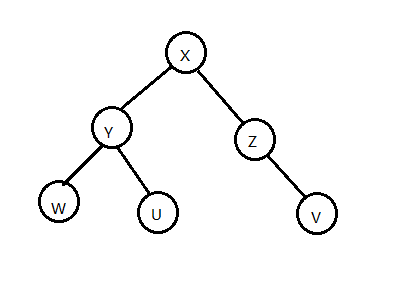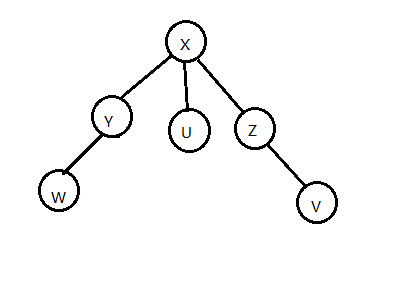\begin{aligned} a &= c + \varphi' - \varphi\\ &= 1+ \sum_{i=1}^{n}r'(i) - \sum_{i=1}^{n}r(i)\\ &= 1 \ + r'(Y)- r(Y)\\ &= 1+ 2-1\\ &= 2\\ \end{aligned}

(假设这一操作实际复杂度是 $1$）

\begin{aligned} \sum_{i=1}^m a_i &= \sum_{i=1}^m c_i + \sum_{i=1}^m(\varphi(x_i)-\varphi(x_{i-1}))\\ &= \sum_{i=1}^m c_i +\varphi(x_n) -\varphi(x_0)\\ \end{aligned} 则有 $$\sum c =\sum a - \varphi(x_n) +\varphi(x_0)$$

$$\sum a \leq \alpha$$

$$\varphi(x_0) - \varphi(x_n) \leq \gamma$$

$$\sum c \leq α + γ$$

(以上只是势能函数应用的一部分，本文对势能函数的应用定义也并不严格)

5.1-2 Splay 复杂度证明

Splay 的时间复杂度要用 势能分析 来证明。

$$\varphi(x)= \sum_{i=1}^{n}r(i)$$

zig / zag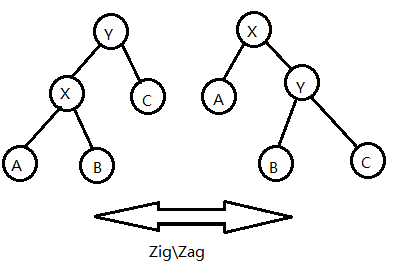zig-zig / zag-zag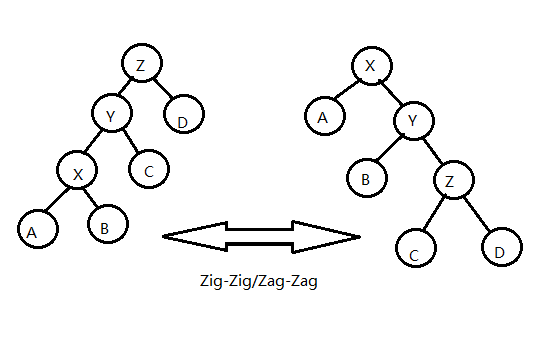$$a = 1 + r'(X) +r'(Y) +r'(Z) -r(X) -r(Y) -r(Z)$$

$$1\leq 2r'(X) - r(X) -r(Z)$$

$$a \leq 3r'(X)- 2r(X) +r'(Z) +r'(Y) -r(Y) -2r(Z)$$

$$r(Y) \ge r(X),r(Z) \ge r'(Z),r(Z) \ge r'(Y)$$

$$0 \leq r(Y) -r(X) +r(Z)-r'(Z)+ r(Z)-r'(Y)$$

$$a \leq 3(r'(X)-r(X))$$

zig-zag / zag-zig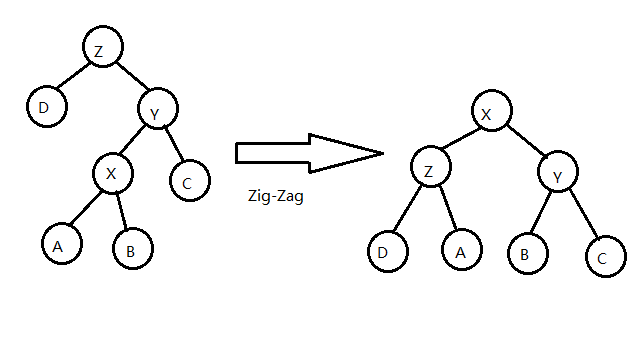$$a = 1 + r'(X) +r'(Y) +r'(Z) -r(X) -r(Y) -r(Z)$$

$$1\leq 2r'(X) - r'(Y) -r'(Z)$$

$$a \leq 3r'(X)- r(X) -r(Y) -r(Z)$$

$$r(Y) \ge r(X),r(Z) \ge r(X)$$

$$0 \leq r(Y) -r(X) +r(Z)-r(X)$$

$$a \leq 3(r'(X)-r(X))$$

总复杂度分析

\begin{aligned} a &\leq 3\sum_{i=1}^m (r(X_i)-r(X_{i-1})) +1\ &\leq 3(r(X_m)-r(X_0)) +1 \end{aligned}

$$a \leq 3\log\ n +1$$

$$\sum_{i=1}^m a_i = \sum_{i=1}^m c_i + \varphi(x_n) -\varphi(x_0)$$

$$\sum_{i=1}^m c_i = \sum_{i=1}^m a_i - \varphi(x_n) +\varphi(x_0)$$

$$\varphi(x_0) -\varphi(x_n) \leq nlogn$$

$$\sum_{i=1}^m c_i \leq 3m \log n+m+n\log n$$

5.2 SATT 时间复杂度证明

$$\varphi(x)= \sum_{i=1}^{n} r(i)$$

$$a \leq 3\log n +1$$

$$a = 1 + r'(\gamma)- 0 \leq \log n +1$$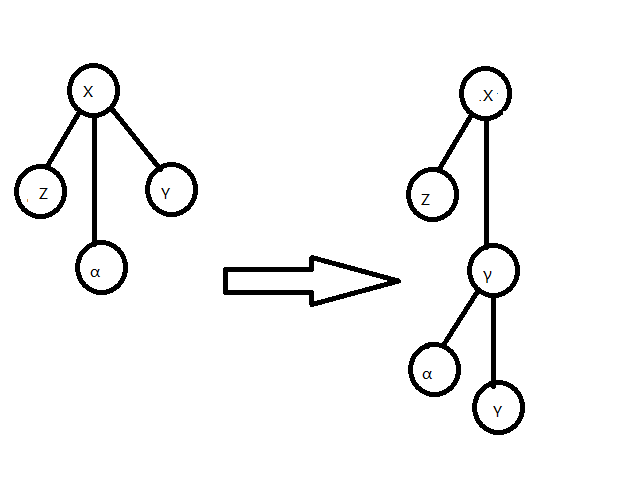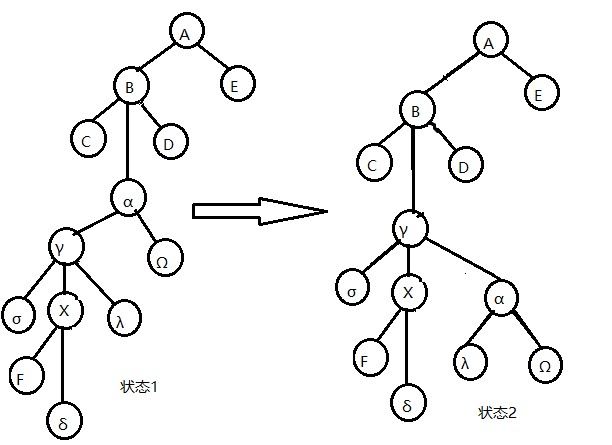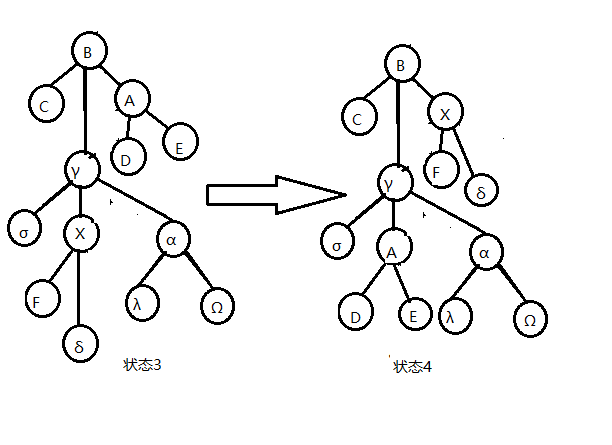$$a \leq 3(r_2(\gamma)- r_1(\gamma))+1$$

$$a \leq 3(r_3(B)- r_2(B))+1$$

$$a = r_4(\gamma) -r_3(\gamma) +1$$

$$a\leq 3r_3(B)+3r_3(B)+3r_2(\gamma)-3r_3(\gamma)-3r_2(B)-3r_1(\gamma)+3$$

$$r_3(\gamma),r_1(\gamma) \ge r_1(X)$$

$$r_3(B),r_2(\gamma) \leq r'(X)\text{ 且 } r_3(B)=r_2(B)$$

$$a\leq 9(r'(X)-r(X))+3$$

$$a \leq 9(r'(x)-r(x))+ 3k + 1$$

$$\sum_{i=1}^m a_i' + \sum_{i=1}^m a_i = \sum_{i=1}^m c_i + \varphi(x_n) -\varphi(x_0)$$

$$\sum_{i=1}^m c_i = \sum_{i=1}^m a_i +\sum_{i=1}^m a_i' - \varphi(x_n) +\varphi(x_0)$$

$$\sum_{i=1}^m c_i \leq \sum_{i=1}^m a_i' + 21m\log n +n\log n +3m$$

$$\sum_{i=1}^m c_i \leq 3(m+n)\log n + 21m\log n +n\log n +4m +n$$

6.例题

CF1192B Dynamic Diameter

void pushup(int x,int op){
if(op==0){
/*是compress node*/
len[x]=len[ls(x)]+len[rs(x)];
diam[x]=maxs[ls(x)]+maxs[rs(x)];
diam[x]=max(diam[x],max(maxs[ls(x)],maxs[rs(x)])+maxs[ms(x)]);
diam[x]=max(diam[x],max(max(diam[ls(x)],diam[rs(x)]),diam[ms(x)]));
maxs[x]=max(maxs[ls(x)],len[ls(x)]+max(maxs[ms(x)],maxs[rs(x)]));
maxs[x]=max(maxs[rs(x)],len[rs(x)]+max(maxs[ms(x)],maxs[ls(x)]));
}
else{
/*是rake node*/
diam[x]=maxs[ls(x)]+maxs[rs(x)];
diam[x]=max(diam[x],maxs[ms(x)]+max(maxs[ls(x)],maxs[rs(x)]));
diam[x]=max(max(diam[x],diam[ms(x)]),max(diam[ls(x)],diam[rs(x)]));
maxs[x]=max(maxs[ms(x)],max(maxs[ls(x)],maxs[rs(x)]));
}
return;
}

void pushrev(int x){
if(!x)return;
r[x]^=1;
swap(ls(x),rs(x));
swap(maxs[x],maxs[x]);
return;
}

[CSP-S2019] 树的重心

SATT 支持动态 $O(\log n)$ 维护树的重心，做到这需要非局部搜索（non-local search）。

void pushup(int x,int op){
if(op==0){
/*是 compress node*/
sum[x]=sum[ls(x)]+sum[rs(x)]+sum[ms(x)]+1;
}
else{
/*是 rake node*/
maxs[x]=max(maxs[ls(x)],max(maxs[rs(x)],sum[ms(x)]));
sum[x]=sum[ls(x)]+sum[rs(x)]+sum[ms(x)];
}
return;
}

1. 比较 簇 $compress(Y)$ 的 $sum$ 值与 簇 $compress(Z)$ 、簇 $A$ 和点 $X$ 的并(我们暂称为簇 $α$ )的 $sum$ 值。若 $compress(Y)$ 的 $sum$ 值大于等于后者，说明至少有一个重心在 $compress(Y)$ 的子树中，我们递归到 $compress(Y)$ 搜索。（如果此处取等，点 $X$ 也是一个重心，需要记录）

2. 比较 簇 $compress(Z)$ 的 $sum$ 值与 簇 $compress(Y)$ 、簇 $A$ 和点 $X$ 的并(我们暂称为簇 $β$ )的 $sum$ 值。若 $compress(Z)$ 的 $sum$ 值大于等于后者，说明至少有一个重心在 $compress(Z)$ 的子树中，我们递归到 $compress(Z)$ 搜索。（如果此处取等，点 $X$ 也是一个重心，需要记录）

3. 比较 点 $x$ 中儿子 rake tree 之中 $sum$ 最大的更小簇（见3-2）的 $sum$ 值与 簇 $compress(Y)$ 、簇 $A$ 、点 $X$ 及其它更小簇的并(我们暂称为簇 $γ$ )的 $sum$ 值，若 那个更小簇 的 $sum$ 值大于等于后者，说明至少有一个重心在 那个更小簇的 子树中，我们递归到它搜索。（如果此处取等，点 $X$ 也是一个重心，需要记录）

4. 若以上比较都不递归，则点 $X$ 一定是一个重心，记录并退出。

void non_local_search(int x,int lv,int rv,int op){

/*lv 和 rv 都是搜索的上一个簇的信息*/

if(!x)return;psd(x,0);
if(op==0){
if(maxs[ms(x)]>=sum[ms(x)]-maxs[ms(x)]+sum[rs(x)]+sum[ls(x)]+lv+1+rv){
if(maxs[ms(x)]==sum[ms(x)]-maxs[ms(x)]+sum[rs(x)]+sum[ls(x)]+lv+1+rv){
if(ans1)ans2=x;else ans1=x;
}
non_local_search(ms(x),sum[ms(x)]-maxs[ms(x)]+sum[rs(x)]+sum[ls(x)]+1+lv+rv,0,1);return;
}
if(ss[rs(x)]+rv>=ss[ms(x)]+ss[ls(x)]+lv+1){
if(ss[rs(x)]+rv==ss[ms(x)]+ss[ls(x)]+lv+1){
if(ans1)ans2=x;else ans1=x;
}
non_local_search(rs(x),sum[ms(x)]+1+sum[ls(x)]+lv,rv,0);return;
}
if(sum[ls(x)]+lv>=sum[ms(x)]+sum[rs(x)]+1+rv){
if(sum[ls(x)]+lv==sum[ms(x)]+sum[rs(x)]+1+rv){
if(ans1)ans2=x;else ans1=x;
}
non_local_search(ls(x),lv,rv+sum[ms(x)]+1+sum[rs(x)],0);return;
}
}
else{
if(maxs[ls(x)]==maxs[x]){non_local_search(ls(x),lv,rv,1);return;}
if(maxs[rs(x)]==maxs[x]){non_local_search(rs(x),lv,rv,1);return;}
non_local_search(ms(x),lv,rv,0);
return;
}
if(ans1)ans2=x;else ans1=x;return;
}

#include<bits/stdc++.h>
#define ls(x) T[x]
#define rs(x) T[x]
#define ms(x) T[x]
#define maxn 1000005
using namespace std;
int s=0;
char a=getchar();
while(!isdigit(a))a=getchar();
while(isdigit(a)){
s=(s<<1)+(s<<3);
s+=a^48;
a=getchar();
}
return s;
}
int T[maxn],tot,n,m,r[maxn],top,st[maxn],f[maxn],maxs[maxn],ss[maxn];
int nnd(){
if(top){top--;return st[top+1];}
else return ++tot;
}
bool isr(int x){return rs(f[x])!=x&&ls(f[x])!=x;}
bool dir(int x){return rs(f[x])==x;}
void psr(int x){if(!x)return;r[x]^=1;swap(ls(x),rs(x));}
void psd(int x,int ty){
if(ty)return;
if(r[x]){psr(ls(x));psr(rs(x));r[x]=0;return;}
}
void psu(int x,int op){
psd(x,op);/*不知道哪没 psd*/
if(op==0){
ss[x]=ss[ls(x)]+ss[rs(x)]+ss[ms(x)]+1;
}
else{
maxs[x]=max(maxs[ls(x)],max(maxs[rs(x)],ss[ms(x)]));
ss[x]=ss[ls(x)]+ss[rs(x)]+ss[ms(x)];
}
return;
}
void upd(int x,int ty){if(!isr(x))upd(f[x],ty);psd(x,ty); }
void stf(int x,int fa,int ty){if(x)f[x]=fa;T[fa][ty]=x;return;}
void rtt(int x,int ty){
int y=f[x],z=f[y],d=dir(x),w=T[x][d^1];
if(z)T[z][ms(z)==y?2:dir(y)]=x;
T[x][d^1]=y;T[y][d]=w;
if(w)f[w]=y;f[y]=x;f[x]=z;
psu(y,ty);psu(x,ty);
}
void spy(int x,int ty,int gl=0){
upd(x,ty);for(int y;y=f[x],(!isr(x))&&y!=gl;rtt(x,ty)){
if(f[y]!=gl&&(!isr(y)))rtt(dir(x)^dir(y)?x:y,ty);
}
}
void cle(int x){ls(x)=ms(x)=rs(x)=ss[x]=r[x]=maxs[x]=f[x]=0;st[++top]=x; }
void del(int x){
stf(ms(x),f[x],1);
if(ls(x)){
int p=ls(x);psd(p,1);while(rs(p))p=rs(p),psd(p,1);
spy(p,1,x);stf(rs(x),p,1);stf(p,f[x],2);psu(p,1);psu(f[x],0);
}
else stf(rs(x),f[x],2);cle(x);
}
void spl(int x){
spy(x,1);int y=f[x];spy(y,0);psd(x,1);
if(rs(y)){
swap(f[ms(x)],f[rs(y)]);swap(ms(x),rs(y));
}
else del(x);psu(x,1);psu(y,0);rtt(rs(y),0);
}
void acs(int x){
spy(x,0);if(rs(x)){
int y=nnd();stf(ms(x),y,0);stf(rs(x),y,2);
rs(x)=0;stf(y,x,2);psu(y,1);psu(x,0);
}
while(f[x])spl(f[x]);
}
void mkr(int x){acs(x);psr(x);}
void epo(int x,int y){mkr(x);acs(y);}
void lnk(int x,int y){acs(x);mkr(y);stf(y,x,1);psu(x,0);}
void cu(int x,int y){epo(x,y);f[x]=ls(y)=0;psu(y,0);}
int ans1,ans2;
void non_local_search(int x,int lv,int rv,int op){
if(!x)return;psd(x,0);
if(op==0){
if(maxs[ms(x)]>=ss[ms(x)]-maxs[ms(x)]+ss[rs(x)]+ss[ls(x)]+lv+1+rv){
if(maxs[ms(x)]==ss[ms(x)]-maxs[ms(x)]+ss[rs(x)]+ss[ls(x)]+lv+1+rv){
if(ans1)ans2=x;else ans1=x;
}
non_local_search(ms(x),ss[ms(x)]-maxs[ms(x)]+ss[rs(x)]+ss[ls(x)]+1+lv+rv,0,1);return;
}
if(ss[rs(x)]+rv>=ss[ms(x)]+ss[ls(x)]+lv+1){
if(ss[rs(x)]+rv==ss[ms(x)]+ss[ls(x)]+lv+1){
if(ans1)ans2=x;else ans1=x;
}
non_local_search(rs(x),ss[ms(x)]+1+ss[ls(x)]+lv,rv,0);return;
}
if(ss[ls(x)]+lv>=ss[ms(x)]+ss[rs(x)]+1+rv){
if(ss[ls(x)]+lv==ss[ms(x)]+ss[rs(x)]+1+rv){
if(ans1)ans2=x;else ans1=x;
}
non_local_search(ls(x),lv,rv+ss[ms(x)]+1+ss[rs(x)],0);return;
}
}
else{
if(maxs[ls(x)]==maxs[x]){non_local_search(ls(x),lv,rv,1);return;}
if(maxs[rs(x)]==maxs[x]){non_local_search(rs(x),lv,rv,1);return;}
non_local_search(ms(x),lv,rv,0);
return;
}
if(ans1)ans2=x;else ans1=x;return;
}
int qu[maxn],qv[maxn];
int main(){
for(I=1;I<=TT;I++){
for(i=1;i<=n;i++)ss[i]=1;
for(i=1;i<=n-1;i++){
}
for(i=1;i<=n-1;i++){
cu(qu[i],qv[i]);
ans1=0;ans2=0;non_local_search(qu[i],0,0,0);
ANS+=ans1+ans2;
if(ans1)acs(ans1);if(ans2)acs(ans2);
ans1=0;ans2=0;non_local_search(qv[i],0,0,0);
ANS+=ans1+ans2;
if(ans1)acs(ans1);if(ans2)acs(ans2);
lnk(qu[i],qv[i]);
}
cout<<ANS<<'\n';
for(i=1;i<=tot;i++)T[i]=T[i]=T[i]=ss[i]=r[i]=maxs[i]=f[i]=0;
tot=top=0;
}
return 0;
}

7.参考资料

1.《Self-Adjusting Top Trees Robert》E. Tarjan， Renato F. Werneck。

2.negiizhao的博客（%%论文哥）

3.zhengrunzhe的sone1题解（%%zhengrunzhe）

KYM_LOVE_MZQ
qp